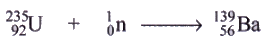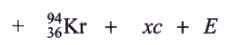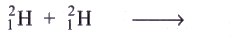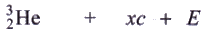Courses

# Solutions of Sources of Energy (Page No- 159) - Physics By Lakhmir Singh, Class 10 Class 10 Notes | EduRev

## Class 10 : Solutions of Sources of Energy (Page No- 159) - Physics By Lakhmir Singh, Class 10 Class 10 Notes | EduRev

The document Solutions of Sources of Energy (Page No- 159) - Physics By Lakhmir Singh, Class 10 Class 10 Notes | EduRev is a part of the Class 10 Course Class 10 Physics Solutions By Lakhmir Singh & Manjit Kaur.
All you need of Class 10 at this link: Class 10

Lakhmir Singh Physics Class 10 Solutions Page No:159

Question 46: A nuclear reaction is represented by the following equation(a) Name the process represented by this equation and describe what takes place in this reaction.
(b) Identify the particle c and the number x of such particles produced in the reaction.
(c) What does E represent ?
(d) Name one installation where the above nuclear reaction is utilised.
(e) What type of bomb is based on similar type of reactions ?

Solution : (a) Nuclear fission; a large nucleus splits into two smaller nuclei with the release of energy, brought about by the absorption of a neutron.
(b) Particles c is neutron; x=3
(c) Energy liberated
(d) Nuclear power station
(e) Atom bomb

Question 47: A nuclear reaction is represented by the equation(a) Name the process represented by this equation and describe what happens during this reaction.
(b) Identify the particle c and the number x of such particles produced in the reaction.
(c) What does £ represent ?
(d) State two conditions under which such a reaction takes place.
(e) What type of nuclear bomb is based on similar reactions ?

Solution : (a) Nuclear fusion; two smaller nuclei combine to form a bigger nucleus with the release of energy, brought about under the conditions of high temperature and pressure.
(b) Particle c is neutron; x=1
(c) Energy liberated
(d) Millions of degree of temperature and millions of pascals of pressure.
(e) Hydrogen bomb.

Question 48: The mass numbers of four elements A, B, C and D are 2, 20, 135 and 235, respectively. Which one of them will be most suitable to make : (i) an atom bomb, and (it)a hydrogen bomb ?

Solution : (i) D(mass number 235)
(ii) A(mass number2)

Question 49: A nuclear power plant is working normally. What would you do if the reactor core suddenly got too hot ?

Solution : We will insert the control rods of boron a little more into the reactor to reduce the rate of nuclear fission process.

Question 50: A nuclear reactor has half the length of all its control rods inserted in graphite. What must be done so that the reactor produces more heat ?

Solution : The control rods should be withdrawn a little more from inside the reactor. This will increase the rate of nuclear fission process and hence more heat.

Question 51: Explain why, in a nuclear reactor, the chain reaction stops if the control rods are fully inserted into the graphite.

Solution : The control rods absorb all the neutrons, stopping the nuclear chain reaction.

Offer running on EduRev: Apply code STAYHOME200 to get INR 200 off on our premium plan EduRev Infinity!

94 docs

,

,

,

,

,

,

,

,

,

,

,

,

,

,

,

,

,

,

,

,

,

,

,

,

;